Home  │ Audio Home Page

Updated March 26, 2011.  See Document History at end for details.

# Low-noise Hybrid Op-amp/Discrete Phono Preamp

### Introduction

Desiring to present a RIAA equalization design example using an operational amplifier, it seems natural to prefer the part with the lowest known noise specification.  Analog Devices AD797 is probably the first device to mind with this qualification.  This part, however, has a dominant pole of about 7.4Hz.  However, its enormous gain and wide bandwidth reduce distortion to levels well below those thought to be audible regardless of the preceding pole specification.  That some say it has a excellent but dark sound is additional confirmation of complete lack of audible distortion.  Because I wanted to have a switchable extra 50kHz zero, I chose the required output buffer following this function to be discrete.  The current-source-biased source-follower buffer is a very low distortion circuit, which I want to add a neutral euphonic distortion profile.  The capacitor is not a detriment in this circuit because it would likely have been needed even with an op-amp output buffer.  This is because the 60dB DC gain and class-a pull up resistor of the first stage would have produced a good bit more offset than a low gain op-amp application.  A capacitor or a dc servo would have been desired regardless.  This circuit also benefits because an operational amplifier RIAA circuit simplifies setting gain and equalization compared to a discrete circuit with its transistor variations and stray impedances.

The schematic and SPICE analyses were created with a limited version of National Instrument's MultiSim offered by Analog Devices to evaluate their products.  As such, all parts available in the program were either Analog Devices components or enough generic parts needed to complete a design.  I maxed the parts count in the schematic below.  Therefore, I could not show paralled standard value capacitors separately.

### Theory of operation

Operational amplifier U1 and its feedback circuit amplify and equalize the input signal to RIAA specifications adding an extra 50kHz zero.  R4 and C4 create a 50kHz pole which switch S1 will use to allow or cancel the extra 50kHz zero.  The output buffer serves to isolate the RC filter and drive the load.  Furthur, the output buffer puts the very high impedance of its JFET's drains between each power supply connection and the signal path.  I intend this to create PSRR (power supply rejection ratio) high enough to reduce power supply concerns in this stage.

### Circuit

Figure 1:  Schematic showing ideal equalization values.  Chosen standard values follow in parts list.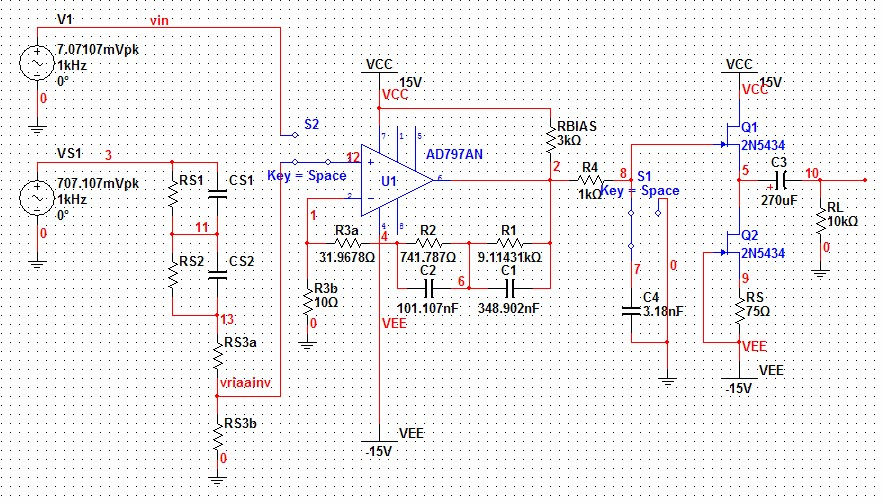Parts List U11 Analog Devices AD797AN C2a4 100nF, 1% Q1, Q22 2N5434  n-ch JFET C2b4 2.2nF, 1% Ri3 47kΩ, 5% RBIAS 3kΩ, 5% Ci3 100pF, 5% R4 1.0kΩ, 1% R1 9.09kΩ, 1% C4a4 3nF, 1% R2 732Ω, 1% C4b4 180pF, 1% R3a 31.6Ω, 1% S1 ½ of DPDT switch or relay (shared with other channel) R3b 10.0Ω, 1% RS 75Ω, 5% C1a4 330nF, 1% C3 270µF, 10% electrolytic C1b4 20nF, 1% RL5 100kΩ, 5%
1A new National Semiconductor operational amplifier LME49990 has specifications nearly identical to Analog Devices AD797, if you desire to investigate an alternative to this part.  It is less hobby friendly because, unlike the AD797, it only available as a surface mount component.
2This part chosen from limited parts list.  I expect you will make substitutions for this part.  See design procedure.
3Ri and Ci, are not shown in the circuit, connect them from input to ground.  Values calculated for your cartidge can be substituted for default values shown.
4A and b subdesignations of parts indicate parallel combinations meant to serve one part function.
5RL in circuit is altered to test distortion under lowest normal load condition.  The actual value is used to properly terminate C3.

### Design Procedure

Calculate RIAA Analysis

See Calculating Non-inverting RIAA Network with Extra Zero for details and derivation of these calculations.

Choose R3b = 10Ω from AD797 datasheet recommendation.
 (1) R3 = R3b 0.2382778 = 10Ω 0.2382778 = 41.9678Ω

 (2) R3a = R3 - R3b = 41.9678Ω - 10Ω = 31.9678Ω

 (3) R2 = R3 × 17.67514356 = 41.9678Ω × 17.67514356 = 741.787Ω

 (4) R1 = R3 × 217.173913 = 41.9678Ω × 217.173913 = 9.11431kΩ

 (5) C1 = TP1 R1 = 3180µs 9.11431kΩ = 348.902nF

 (6) C2 = TP2 R2 = 75µs 741.787Ω = 101.107nF

Now calculate extra-zero switch out circuit:

Choose R4 = 1kΩ and calculate for C4:
 (7) C4 = TZ2 R4 = 3.18µs 1kΩ = 3.18nF

Calculate Class-A Biasing

Here the concept is that applying the supply voltage through a voltage divider comprising RBIAS and the lowest load resistance expected should result in a voltage across the load exceeding the maximum expected peak signal.  Assuming a minimum load of 10kΩ and a peak signal of 2.828V calculate:
 (8) RBIAS-MAX = 10kΩ × 15V - 2.828V 15V = 8.11467kΩ
For the purpose of allowing headroom calculate RBIAS for a reasonable bias within the selected op amp's current capacity.  Choose 5mA bias and calculate RBIAS:
 (9) RBIAS-MIN = 15V 5mA = 3kΩ
Choose lower of two RBIAS calculations:
 (10) RBIAS = 3kΩ
Connect this bias to positive supply to have any dc offset contribution pull output capacitor in the same direction as the output buffer does.  This is to prevent capacitor bias uncertainty in the unlikely event that the class-a bias produces an offset near to the operating value of VGS.

Output Buffer Design

The limits of the trial edition of MultiSim limited my choice of JFET's.  It is fortunate then that this choice is not critical.  As for noise the op-amp has amplified its noise enough to make that of the output less critical.  Consider that 1nV/√Hz amplified by about 100 gain at 1kHz is 100nV/√Hz.  A common 2N3819 would be a good choice as would others in this respect.  It is better to prioritize the maximization of gfs in order to reduce buffer stage distortion.  To this end, an LSK170 would be a best known choice due to high kn and gfs, with the lowest possible noise for a bonus.  The best compromise choice given me by MultiSim was a 2N5434 with a kn of 22mA/V2.  Even this part is now obsolete.  Since you would be faced with component variations on a part of your own choice, I suggest you choose a value of RS by trial and error to get a bias current within your JFETs specifications.  You should not match Q1 and Q2.  Rather, because Q1 will operate freer if it is not pushed to its current limit, you should choose Q1 to have a higher gfs than Q2.  To this end, try both transistors in the Q2 position and place the one there that gives the lowest bias current through Q2.  Since this aspect is experimental, calculating RS may be overengineering.  A RS corresponding to the JFET's rated gfs would be a good starting point for a midpoint bias, i.e.:
 (11) RS = 1 gfs = 1 17mS = 58.8235Ω
or alternatively setting RS to ratio of cutoff voltage to maximum current for another approximate midpoint bias:
 (12) RS = |VT| |IDSS| = 2.5V 30mA = 83.3333Ω
If you must make an exact calculation from a chosen ID, the following was derived from the FET equation by circuit analysis:
 (13) RS = VT - sqrt(ID/kn) ID
Choose RS = 75Ω as median of two estimates.

Calculate output coupling capacitor to give 1Hz highpass pole with a 600Ω load knowing that the pole frequency will be much lower with a more reasonable load of 10kΩ:

 (14) C3 = 1 2π × RL-MIN × fhp = 1 2π × 600Ω × 1Hz = 265.2582385µF
Round up to next standard value C3 = 270µF

### Spice Simulation

SPICE model for ideal circuit.
SPICE model for final circuit.

Magnitude in all Bode plots and Voltage in distortion plots are in units of dB.

 Figure 2:  Bode and phase plots from ideal circuit before choosing standard values. Cyan plot shows 50kHz zero switched in and magenta plot out.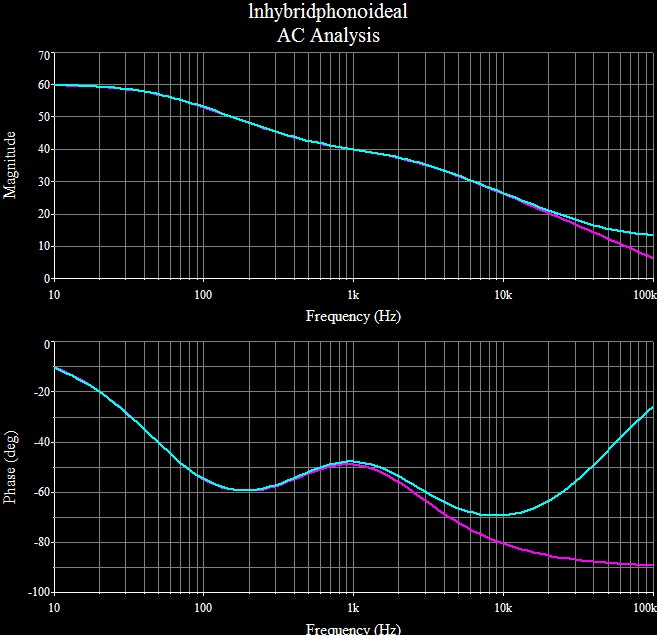Figure 3:  Same plots with circuit driven by RIAA inverse preequalizer with extra pole at 50kHz. Cyan plot shows 50kHz zero switched in and magenta plot out.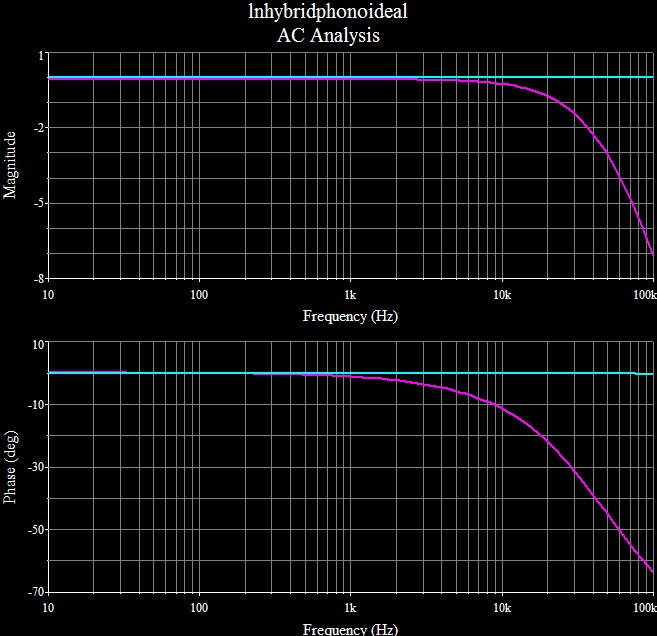Juggle ac analysis in SPICE to choose standard values:

 Figure 4:  Preequalized bode plot with chosen standard values. Cyan plot shows 50kHz zero switched in and magenta plot out.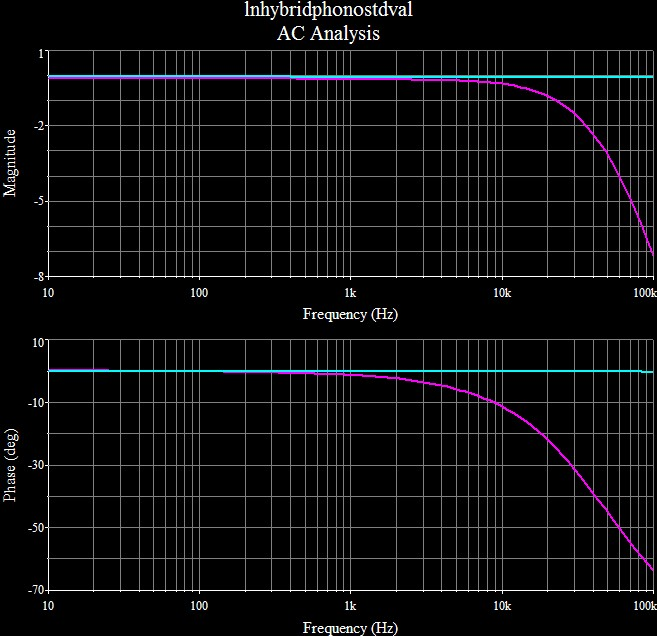All Distortion analyses done with RIAA preequalization to give signal levels the correct frequency balance. Figure 5:  Fourier analysis shows harmonic distribution of 0.0022818% distortion of 1kHz signal.  Note dominance of second harmonic and to a lesser extent the other even harmonics.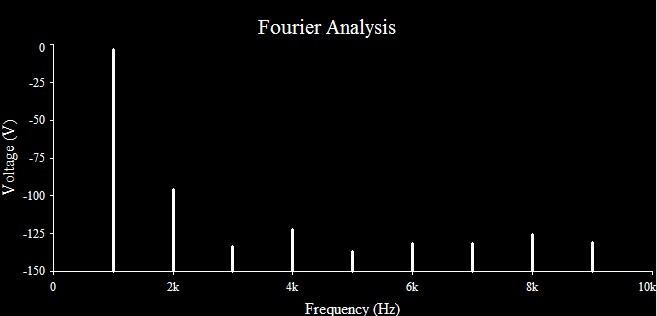Figure 6:  2rd harmonic distortion plot magenta plot shows op-amp distortion rising with frequency and cyan plot shows it masked by frequency-flat distortion profile of output stage.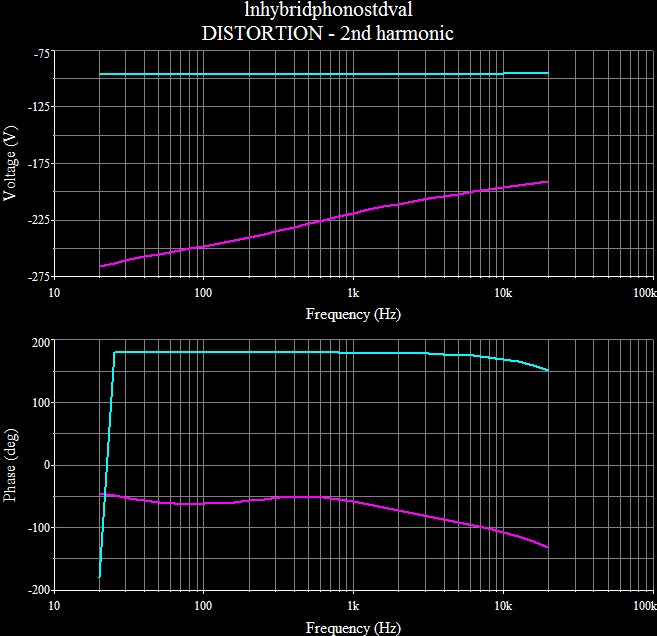Figure 7:  3rd harmonic distortion plot magenta plot shows op-amp distortion rising with frequency and cyan plot shows it masked by frequency-flat distortion profile of output stage.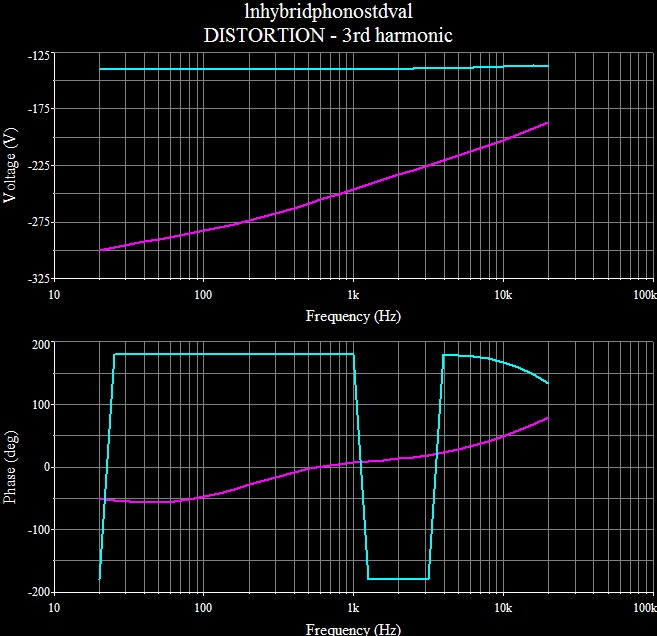Miscellaneous Spice Results SNR = 99.7334dB relative to 1VRMS output. AC analyses with ac sources in series with power supplies showed high PSRR in output buffer:  PSRR measured 91dB relative to VCC and higher relative to VEE.  I did this investigation at the outset to verify my presumptions about the buffer's PSRR and do not remember the second figure exactly.  This suggests that a power supply sufficient for the first stage will suffice for the buffer as well.